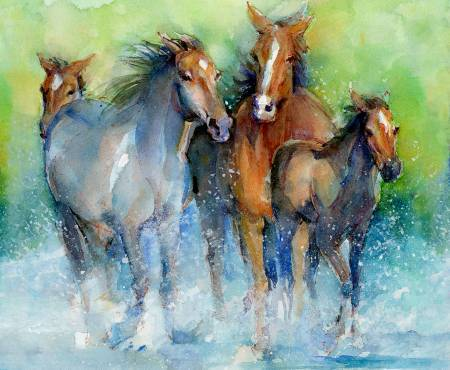# New Fabrics!

Awesome horse panel!
New white on whites!
New greens and pinks!

 table div table+table+table+table div table{width:100%;padding:0}table div table+table+table+table div table img{width:96.23%;padding:0;float:none}table div table+table+table+table div table td{width:100%;padding:0 1.88% 18px}/* styles */# Planetary Mission

If you know a science lover, you need to make them something from Planetary Mission!
Quilts, pillows, even backpacks or bags - they will love it!

 table div table+table+table+table+table+table+table div table{width:100%;padding:0}table div table+table+table+table+table+table+table div table img{width:96.23%;padding:0;float:none}table div table+table+table+table+table+table+table div table td{width:100%;padding:0 1.88% 18px}/* styles */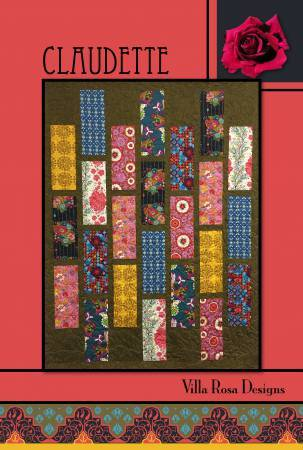# New Villa Rosa Patterns!

Get out your favorite fat quarters, pick a Villa Rosa pattern and start sewing! These are the post card patterns - simple designs to show off your fabrics and all these use fat quarters!

 table div table+table+table+table+table+table+table+table+table+table div table{width:100%;padding:0}table div table+table+table+table+table+table+table+table+table+table div table img{width:96.23%;padding:0;float:none}table div table+table+table+table+table+table+table+table+table+table div table td{width:100%;padding:0 1.88% 18px}/* styles */## Hey Tula Pink Fans!

 /* styles */ Just so you know.... We have all of Lineworks ordred, including the wideback and precuts! Free Spirit is expecting to ship the last week of October, so be sure to watch our Sunday Soiree FB Live on November 1! (I tried for half an hour to get a photo to upload, but my computer wasn't having any of it - sorry!)
 table div table+table+table+table+table+table+table+table+table+table+table+table+table div table{width:100%;padding:0}table div table+table+table+table+table+table+table+table+table+table+table+table+table div table img{width:96.23%;padding:0;float:none}table div table+table+table+table+table+table+table+table+table+table+table+table+table div table td{width:100%;padding:0 1.88% 18px}/* styles */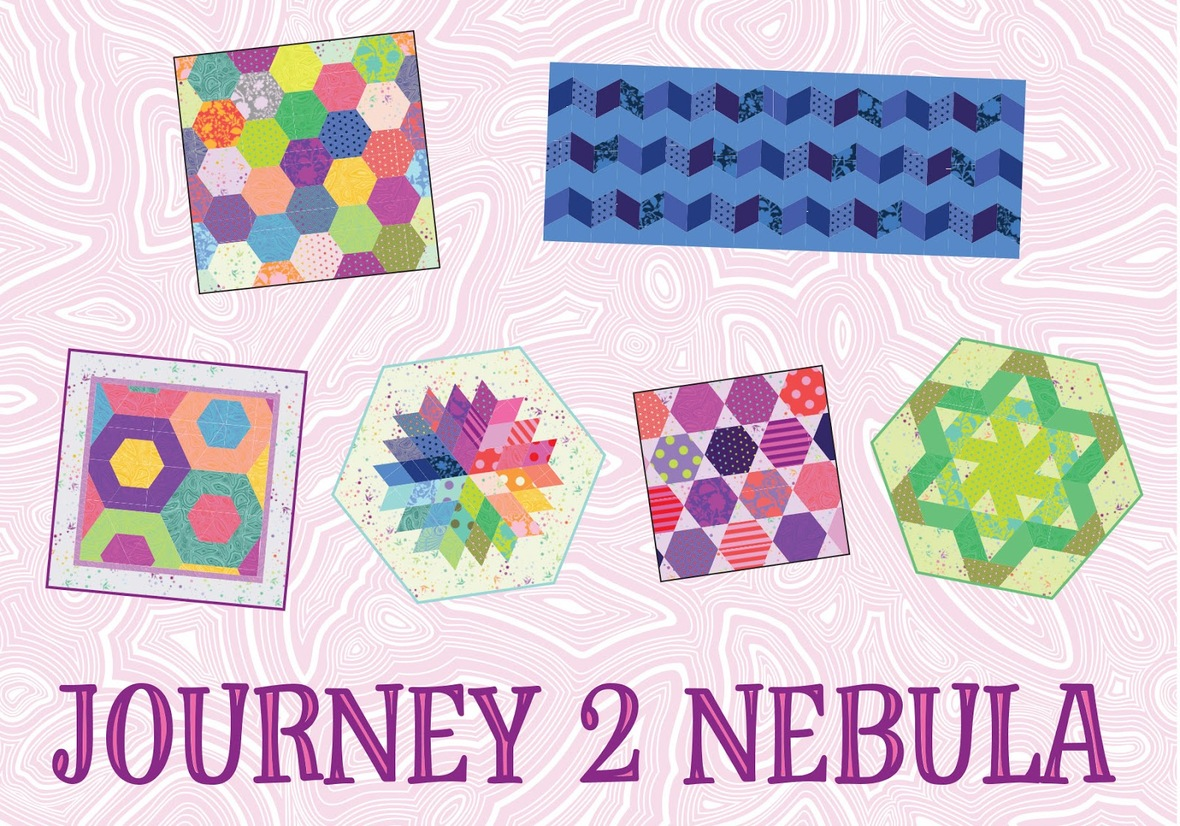# Journey 2 Nebula

Nebula is the block of the month by Jaybird Quilts using Tula fabrics that starts in January.
Whether or not you signed up for Nebula (and we are full right now), the Journey 2 Nebula is going to be fun!
Designer Julie Herman of Jaybird Quilts is hosting a quilt along with six small projects to become familiar with the tools used in Nebula.
Patterns have been delayed, so we are a bit behind Julie's schedule, but you can watch the videos anytime.
Once I am able to ship the patterns and rulers, we will have a Cotton Patch FB Group to share tips and photos.

 table div table+table+table+table+table+table+table+table+table+table+table+table+table+table+table+table+table div table{width:100%;padding:0}table div table+table+table+table+table+table+table+table+table+table+table+table+table+table+table+table+table div table img{width:96.23%;padding:0;float:none}table div table+table+table+table+table+table+table+table+table+table+table+table+table+table+table+table+table div table td{width:100%;padding:0 1.88% 18px}/* styles */# Take a Class Online!

How does this work you may be wondering?
Your "classroom" will be on Facebook, in a private group that you will be invited to join after registering.

Classes are recorded, you will have access to them 24/7 in the private Facebook group and can view them as many times as you like, whenever you like!

# ONLINE CLASS BONUS!

## Every quilt class will have a bonus video on borders and bindings!

 table div table+table+table+table+table+table+table+table+table+table+table+table+table+table+table+table+table+table+table div table{width:100%;padding:0}table div table+table+table+table+table+table+table+table+table+table+table+table+table+table+table+table+table+table+table div table img{width:96.23%;padding:0;float:none}table div table+table+table+table+table+table+table+table+table+table+table+table+table+table+table+table+table+table+table div table td{width:100%;padding:0 1.88% 18px}/* styles */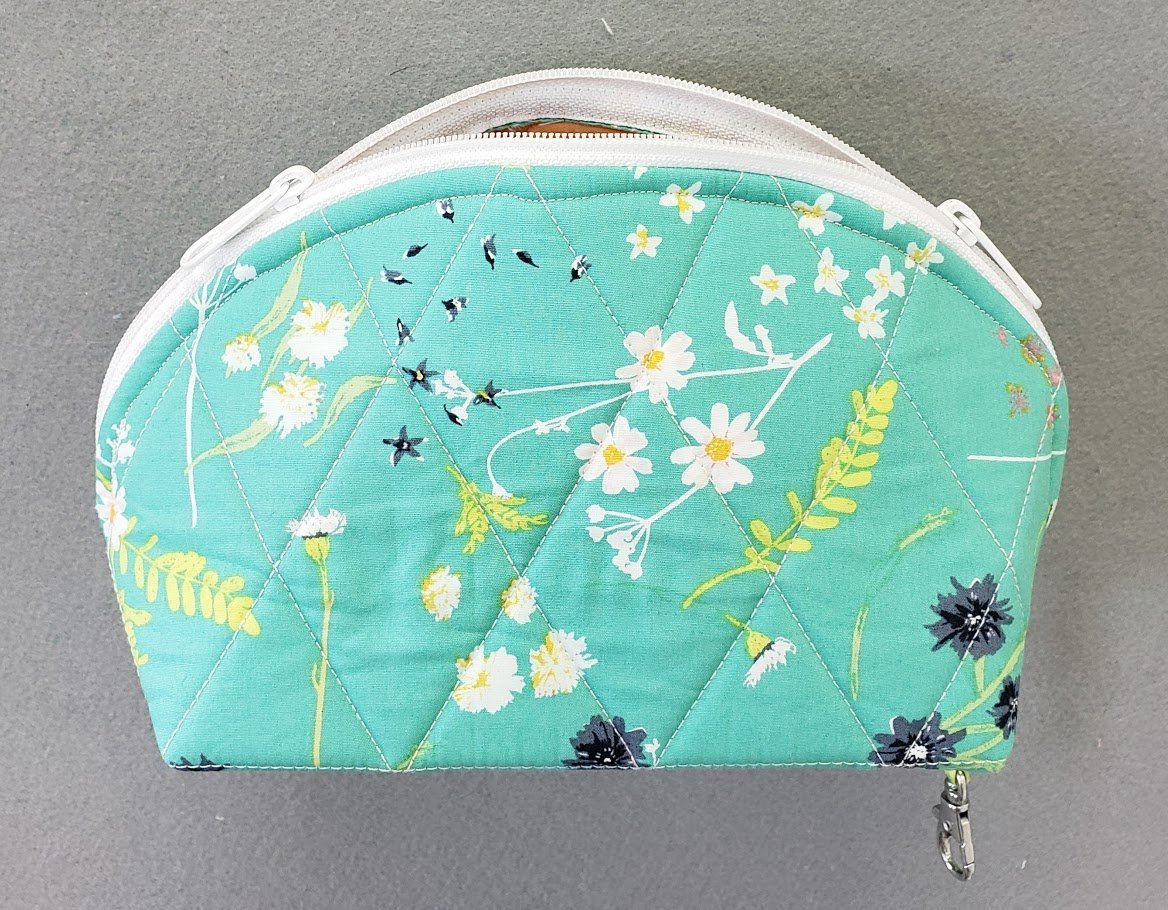# Introducing the latest ONLINE Class!

Clam Up Pouches!
You will learn not to fear zippers!
And with a few basic quilting tricks you will be ready to play with your new favorite bag.

 table div table+table+table+table+table+table+table+table+table+table+table+table+table+table+table+table+table+table+table+table+table+table div table{width:100%;padding:0}table div table+table+table+table+table+table+table+table+table+table+table+table+table+table+table+table+table+table+table+table+table+table div table img{width:96.23%;padding:0;float:none}table div table+table+table+table+table+table+table+table+table+table+table+table+table+table+table+table+table+table+table+table+table+table div table td{width:100%;padding:0 1.88% 18px}/* styles */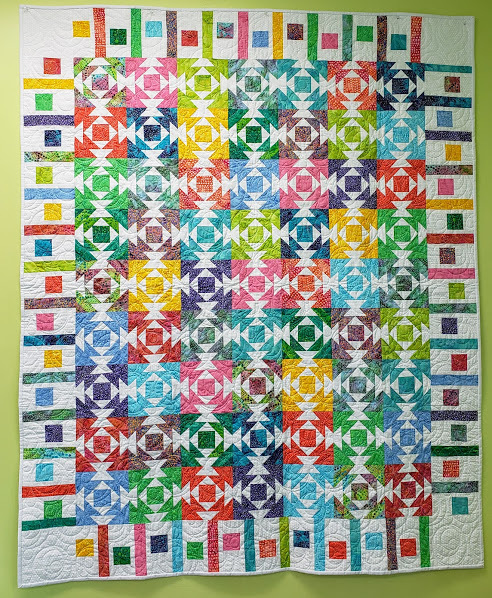# Bubbles Pineapple Quilt!

If you have ever wanted to make a pineapple quilt but thought it would be too difficult or if you ever tried to make one and it came out wonky this is the online course made for you.

 table div table+table+table+table+table+table+table+table+table+table+table+table+table+table+table+table+table+table+table+table+table+table+table+table+table div table{width:100%;padding:0}table div table+table+table+table+table+table+table+table+table+table+table+table+table+table+table+table+table+table+table+table+table+table+table+table+table div table img{width:96.23%;padding:0;float:none}table div table+table+table+table+table+table+table+table+table+table+table+table+table+table+table+table+table+table+table+table+table+table+table+table+table div table td{width:100%;padding:0 1.88% 18px}/* styles */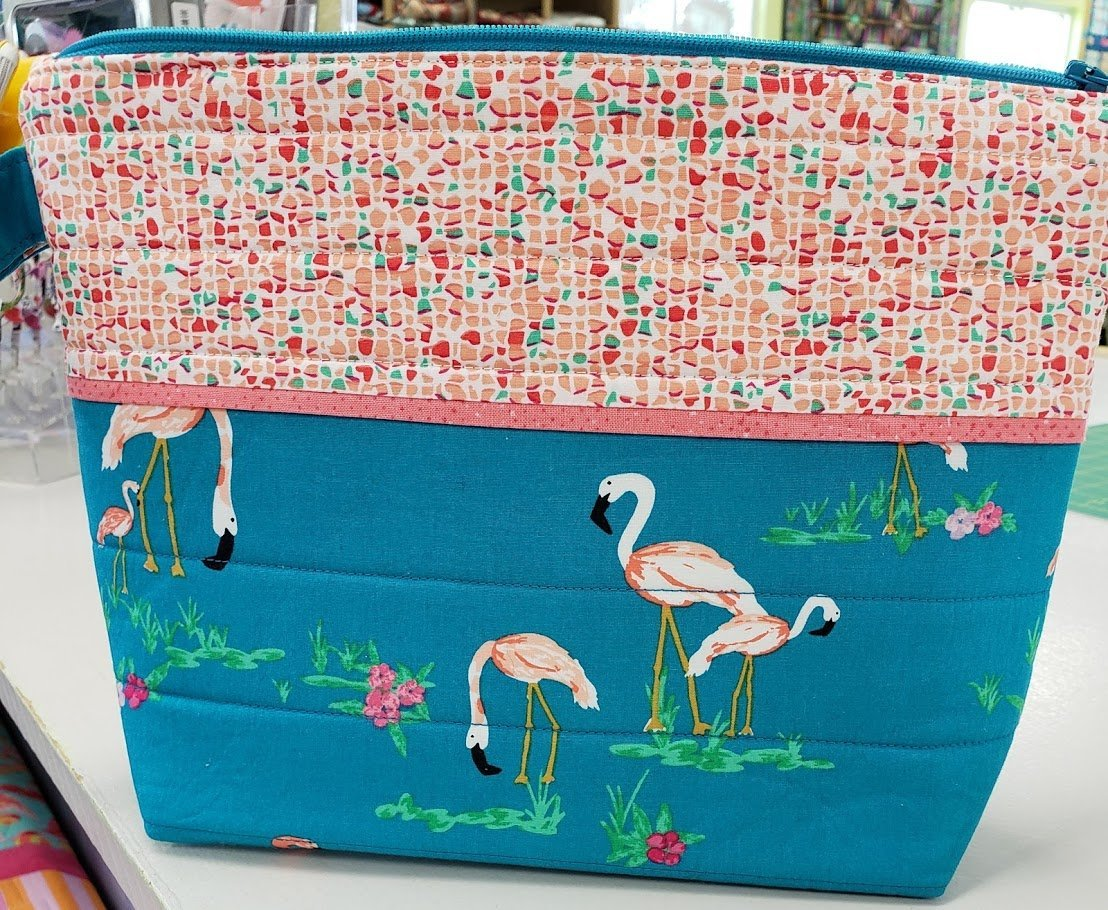# Sew Essential ONLINE Class!

The Sew Essential Bag is a great bag to organize your sewing supplies!
Margaret promises after this class you will be cured of Zipper-phobia!

 table div table+table+table+table+table+table+table+table+table+table+table+table+table+table+table+table+table+table+table+table+table+table+table+table+table+table+table+table div table{width:100%;padding:0}table div table+table+table+table+table+table+table+table+table+table+table+table+table+table+table+table+table+table+table+table+table+table+table+table+table+table+table+table div table img{width:96.23%;padding:0;float:none}table div table+table+table+table+table+table+table+table+table+table+table+table+table+table+table+table+table+table+table+table+table+table+table+table+table+table+table+table div table td{width:100%;padding:0 1.88% 18px}/* styles */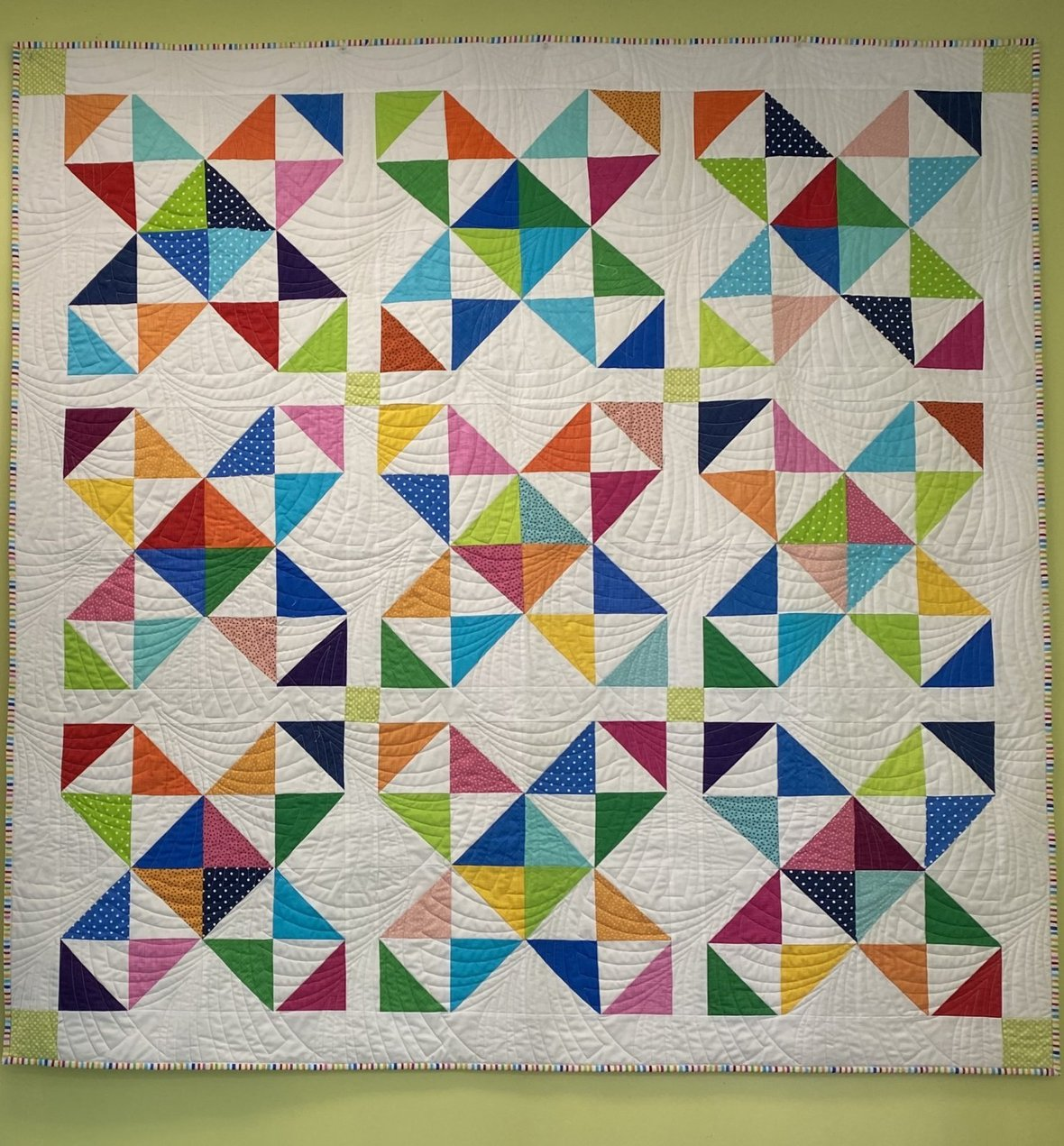# Twinkle ONLINE Class!

Marlyn has tips and tricks so you can make your own Twinkle quilt!
You'll be a whiz at half square triangles!

 table div table+table+table+table+table+table+table+table+table+table+table+table+table+table+table+table+table+table+table+table+table+table+table+table+table+table+table+table+table+table+table div table{width:100%;padding:0}table div table+table+table+table+table+table+table+table+table+table+table+table+table+table+table+table+table+table+table+table+table+table+table+table+table+table+table+table+table+table+table div table img{width:96.23%;padding:0;float:none}table div table+table+table+table+table+table+table+table+table+table+table+table+table+table+table+table+table+table+table+table+table+table+table+table+table+table+table+table+table+table+table div table td{width:100%;padding:0 1.88% 18px}/* styles */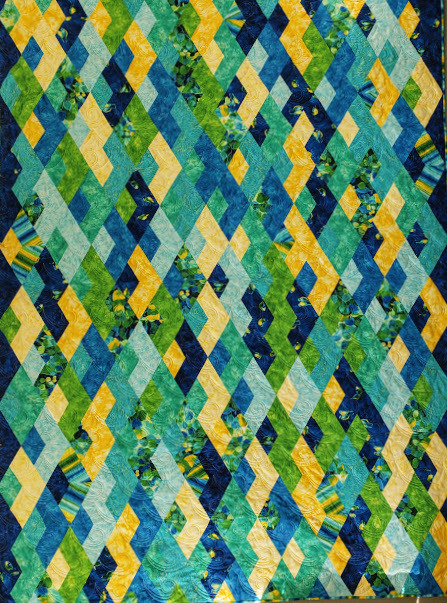# Boomerang ONLINE Class!

Cotton Patch is stepping into the virtual world with our first online class - Boomerang which will first be available on August 8

This fun and challenging quilt will have you inspired to play with your color wheel.

Boomerang is a true skill builder for confident beginners to advanced sewers.

 table div table+table+table+table+table+table+table+table+table+table+table+table+table+table+table+table+table+table+table+table+table+table+table+table+table+table+table+table+table+table+table+table+table+table div table{width:100%;padding:0}table div table+table+table+table+table+table+table+table+table+table+table+table+table+table+table+table+table+table+table+table+table+table+table+table+table+table+table+table+table+table+table+table+table+table div table img{width:96.23%;padding:0;float:none}table div table+table+table+table+table+table+table+table+table+table+table+table+table+table+table+table+table+table+table+table+table+table+table+table+table+table+table+table+table+table+table+table+table+table div table td{width:100%;padding:0 1.88% 18px}/* styles */# Quilter's Trek!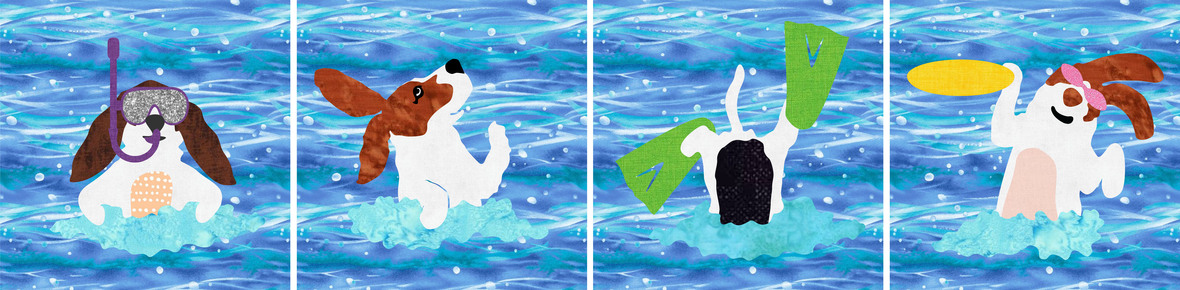/* styles */ It's the Dippin' Dawgs! The theme this year is the color blue Shops were to design 4 blocks that finish at 9" square Blocks can be put together as a row, a square, or used individually. So Freddie, Maggie, Stanley and Lulu are having a day at the beach!
 table div table+table+table+table+table+table+table+table+table+table+table+table+table+table+table+table+table+table+table+table+table+table+table+table+table+table+table+table+table+table+table+table+table+table+table+table+table+table+table div table{width:100%;padding:0}table div table+table+table+table+table+table+table+table+table+table+table+table+table+table+table+table+table+table+table+table+table+table+table+table+table+table+table+table+table+table+table+table+table+table+table+table+table+table+table div table img{width:96.23%;padding:0;float:none}table div table+table+table+table+table+table+table+table+table+table+table+table+table+table+table+table+table+table+table+table+table+table+table+table+table+table+table+table+table+table+table+table+table+table+table+table+table+table+table div table td{width:100%;padding:0 1.88% 18px}/* styles */# FB Live is the place to be Sunday at 7pm!

You will be the 1st to see new fabrics, notions, kits and other great things that you need to know about!

## We put together fun kits that often sell out that very night - you don't want to miss out!

There will be prizes, live demos and special pricing!

You will be able to purchase during the Live Broadcast and we can ship - sort of like Stuff Your Stocking but all in one night.
If you miss the FB Live - not to worry!

## You can view the replay on our FB Page AND you can still purchase at the special prices until 3pm Monday!

We will go "live" at 6:55 and see what the hounds are up to, then
start the Soiree at 7pm (Eastern)

## Join me for a fun evening!

 table div table+table+table+table+table+table+table+table+table+table+table+table+table+table+table+table+table+table+table+table+table+table+table+table+table+table+table+table+table+table+table+table+table+table+table+table+table+table+table+table+table+table+table div table{width:100%;padding:0}table div table+table+table+table+table+table+table+table+table+table+table+table+table+table+table+table+table+table+table+table+table+table+table+table+table+table+table+table+table+table+table+table+table+table+table+table+table+table+table+table+table+table+table div table img{width:96.23%;padding:0;float:none}table div table+table+table+table+table+table+table+table+table+table+table+table+table+table+table+table+table+table+table+table+table+table+table+table+table+table+table+table+table+table+table+table+table+table+table+table+table+table+table+table+table+table+table div table td{width:100%;padding:0 1.88% 18px}/* styles */# Meet me in the Club House!

Cotton Patch Club House is a new FaceBook group and we want you to be a part of it!
You will find:
An online community for friends of Cotton Patch Quilt Shop!
A place to chat and share photos of your projects.
A place to ask quilty questions, share tips and demos
A place for you to find sneak peeks and early access to items on our FaceBook Live Sunday Soiree!

 table div table+table+table+table+table+table+table+table+table+table+table+table+table+table+table+table+table+table+table+table+table+table+table+table+table+table+table+table+table+table+table+table+table+table+table+table+table+table+table+table+table+table+table+table+table+table div table{width:100%;padding:0}table div table+table+table+table+table+table+table+table+table+table+table+table+table+table+table+table+table+table+table+table+table+table+table+table+table+table+table+table+table+table+table+table+table+table+table+table+table+table+table+table+table+table+table+table+table+table div table img{width:96.23%;padding:0;float:none}table div table+table+table+table+table+table+table+table+table+table+table+table+table+table+table+table+table+table+table+table+table+table+table+table+table+table+table+table+table+table+table+table+table+table+table+table+table+table+table+table+table+table+table+table+table+table div table td{width:100%;padding:0 1.88% 18px}/* styles *//* styles */ Although the Stay at Home Order has been lifted in Florida, the storefront at Cotton Patch will remained closed to shopping for now. An opening date is still to be determined. Our website is open 24/7 and we are filling orders for curbside pickup or shipping right to your door! To best serve you, all orders should be placed through the website and not over the phone. Payment on the website is more secure than reading your credit card numbers over the phone and less chance for errors on items/quantity. Curbside pickup is available 10am - 2pm Tuesday through Saturday. You will get an email telling you when you order is ready for pickup. Call us from the parking lot and we will bring your purchases out to you. Thank you for your continued support with online orders and curbside pickup - we appreciate your business! We miss your smiling faces and look forward to welcoming you back soon! Laura
 table div table+table+table+table+table+table+table+table+table+table+table+table+table+table+table+table+table+table+table+table+table+table+table+table+table+table+table+table+table+table+table+table+table+table+table+table+table+table+table+table+table+table+table+table+table+table+table+table div table{width:100%;padding:0}table div table+table+table+table+table+table+table+table+table+table+table+table+table+table+table+table+table+table+table+table+table+table+table+table+table+table+table+table+table+table+table+table+table+table+table+table+table+table+table+table+table+table+table+table+table+table+table+table div table img{width:96.23%;padding:0;float:none}table div table+table+table+table+table+table+table+table+table+table+table+table+table+table+table+table+table+table+table+table+table+table+table+table+table+table+table+table+table+table+table+table+table+table+table+table+table+table+table+table+table+table+table+table+table+table+table+table div table td{width:100%;padding:0 1.88% 18px}/* styles *//* styles */ Freddie is helping Julie with some computer work, and you know he's thinking that he's getting paid in treats!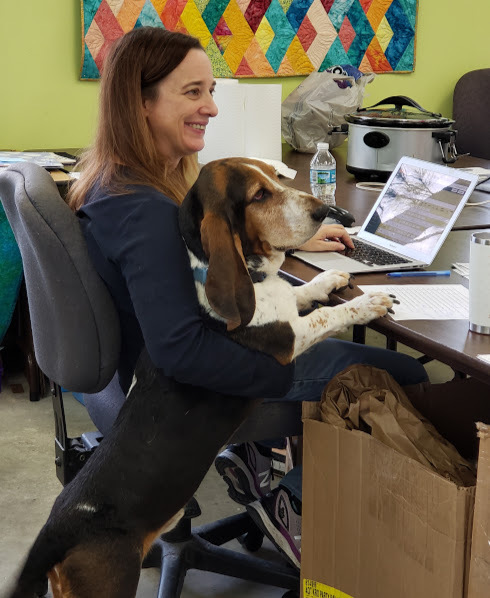Like   Pin   +1